# Cone

Into rotating cone with dimensions r = 8 cm and h = 8 cm incribe cylinder with maximum volume so that the cylinder axis is perpendicular to the axis of the cone. Determine the dimensions of the cylinder.

Result

r =  2.67 cm
h =  5.33 cm

#### Solution:Leave us a comment of example and its solution (i.e. if it is still somewhat unclear...):Be the first to comment!## Next similar examples:

1. Jar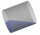From the cylinder shaped jar after tilting spilled water so that the bottom of the jar reaches the water level accurately into half of the base. Height of jar h = 7 cm and a jar diameter D is 12 cm. How to calculate how much water remains in the jar?
2. Owner of the company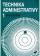The owner of the company with extensive administrative activity wants to sell the obsolete 3 machines at the price of CZK 1000 per 1 machine with a six-month warranty period. If the machine breaks down during this period, the owner will return the employe
3. Balls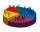We have n identical balls (numbered 1-n) is selected without replacement. Determine 1) The probability that at least one tensile strength number coincides with the number of balls? 2) Determine the mean and variance of the number of balls, which coincides.
4. Median and modusRadka made 50 throws with a dice. The table saw fit individual dice's wall frequency: Wall Number: 1 2 3 4 5 6 frequency: 8 7 5 11 6 13 Calculate the modus and median of the wall numbers that Radka fell.
5. Cylindrical containerAn open-topped cylindrical container has a volume of V = 3140 cm3. Find the cylinder dimensions (radius of base r, height v) so that the least material is needed to form the container.
6. Paper boxHard rectangular paper has dimensions of 60 cm and 28 cm. The corners are cut off equal squares and the residue was bent to form an open box. How long must be side of the squares to be the largest volume of the box?
7. Statue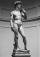On the pedestal high 4 m is statue 2.7 m high. At what distance from the statue must observer stand to see it in maximum viewing angle? Distance from the eye of the observer from the ground is 1.7 m.
8. Curve and lineThe equation of a curve C is y=2x² -8x+9 and the equation of a line L is x+ y=3 (1) Find the x co-ordinates of the points of intersection of L and C. (2) Show that one of these points is also the stationary point of C?
9. Fall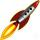The body was thrown vertically upward at speed v0 = 79 m/s. Body height versus time describe equation ?. What is the maximum height body reach?
10. Theorem proveWe want to prove the sentence: If the natural number n is divisible by six, then n is divisible by three. From what assumption we started?
11. Derivation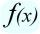Exists a function whose derivation is the same function?
12. The determinant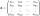The determinant of the unit matrix equals 7. Check how many rows the A matrix contains.
13. Inverse matrixFind how many times is the larger determinant is the matrix A, which equals 9 as the determinant of its inverse matrix.
14. Minimum of sum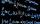Find a positive number that the sum of the number and its inverted value was minimal.
15. AS sequenceIn an arithmetic sequence is given the difference d = -3 and a71 = 455. a) Determine the value of a62 b) Determine the sum of 71 members.
16. Six termsFind the first six terms of the sequence a1 = -3, an = 2 * an-1
17. RootsDetermine the quadratic equation absolute coefficient q, that the equation has a real double root and the root x calculate: ?